# Compact orientable surface

## Classification

Further information: classification of compact orientable surfaces

These are classified by the nonnegative integers. In other words, there is a correspondence:

Nonnegative integers$\leftrightarrow$ Homeomorphism classes of compact orientable surfaces

The correspondence, in the forward direction, is as follows: given a nonnegative integer$g$, the corresponding compact orientable surface, called the surface of genus$g$, is defined as a connected sum of$g$ copies of the 2-torus. Two special cases are of note: for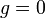$g = 0$, we take the corresponding surface to be the 2-sphere, and for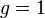$g = 1$, we take the corresponding surface to be the 2-torus. After that, each time we increment$g$ by$1$, we take the connected sum with a new 2-torus.

The surface of genus$g$ is sometimes denoted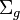$\Sigma_g$,$S_g$ or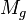$M_g$.

Pictorially, the surface of genus$g$ can be embedded in$\R^3$ with as many holes as the genus.

## Particular cases

Value of genus$g$ Surface
0 2-sphere
1 2-torus
2 genus two surface

## Algebraic topology

### Homology

Further information: homology of compact orientable surfaces

The homology groups of the surface with genus$g$ are given as follows: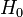$H_0$ and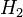$H_2$ are both$\mathbb{Z}$, and$H_1$ is isomorphic to$\mathbb{Z}^{2g}$.

In particular, the Betti numbers are$b_0 = 1, b_1 = 2g, b_2 = 1$, the Poincare polynomial is$1 + 2gx + x^2$, and the Euler characteristic is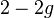$2 - 2g$.

We see from this that the surfaces of genus$g$ are all in different homotopy classes and are in fact not even homology-equivalent. We can in fact recover the genus of a compact orientable surface simply from its Euler characteristic, by$g = 1 - (\chi/2)$.

### Cohomology

Further information: cohomology of compact orientable surfaces

### Homotopy

Further information: homotopy of compact orientable surfaces

## Operations

### Connected sum

The connected sum of a surface with genus$g_1$ and a surface with genus$g_2$ is a surface with genus$g_1 + g_2$. If the Euler characteristics of the surfaces are$\chi_1$ and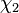$\chi_2$ respectively, the Euler characteristic of the connected sum is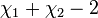$\chi_1 + \chi_2 - 2$.

Thus, the set of homeomorphism classes of compact orientable surfaces under connected sum is isomorphic to the monoid of nonnegative integers under addition.

### Covering spaces

Suppose$S_g$ is a surface of genus$g$,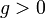$g > 0$. Then, it turns out that for any finite group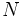$N$ of order$n$, there exists a regular covering map with base$S_g$ and degree$d$ such that the group of deck transformations for the covering map is$N$. The covering space for this map must also be a compact orientable surface, and have genus$h$ for some$h$.$g,h,n$ are related as follows: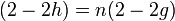$(2 - 2h) = n(2 - 2g)$

or, upon simplification: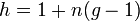$h = 1 + n(g - 1)$

The justification is as follows: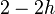$2 - 2h$ and$2 - 2g$ are respectively the Euler characteristics of the compact orientable surfaces, and Euler characteristic of covering space is product of degree of covering and Euler characteristic of base.